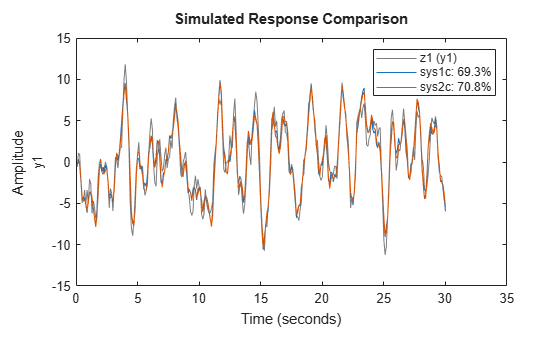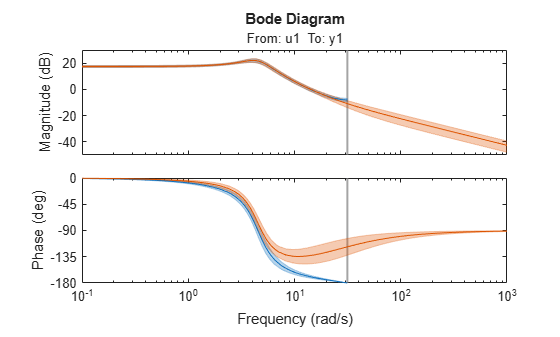# d2c

Convert model from discrete to continuous time

## Syntax

``sysc = d2c(sysd)``
``sysc = d2c(sysd,method)``
``sysc = d2c(sysd,opts)``
``````[sysc,G] = d2c(___)``````

## Description

example

````sysc = d2c(sysd)` converts a the discrete-time dynamic system model `sysd` to a continuous-time model using zero-order hold on the inputs.```

example

````sysc = d2c(sysd,method)` specifies the conversion method.```

example

````sysc = d2c(sysd,opts)` specifies conversion options for the discretization.```
``````[sysc,G] = d2c(___)```, where `sysd` is a state-space model, returns a matrix `G` that maps the states `xd[k]` of the discrete-time state-space model to the states `xc(t)` of `sysc`.```

## Examples

collapse all

Create the following discrete-time transfer function:

`$H\left(z\right)=\frac{z-1}{{z}^{2}+z+0.3}$`

`H = tf([1 -1],[1 1 0.3],0.1);`

The sample time of the model is ${T}_{s}=0.1s$.

Derive a continuous-time, zero-order-hold equivalent model.

`Hc = d2c(H)`
```Hc = 121.7 s + 1.758e-12 --------------------- s^2 + 12.04 s + 776.7 Continuous-time transfer function. ```

Discretize the resulting model, `Hc`, with the default zero-order hold method and sample time 0.1s to return the original discrete model, `H`.

`c2d(Hc,0.1)`
```ans = z - 1 ------------- z^2 + z + 0.3 Sample time: 0.1 seconds Discrete-time transfer function. ```

Use the Tustin approximation method to convert `H` to a continuous time model.

`Hc2 = d2c(H,'tustin');`

Discretize the resulting model, Hc2, to get back the original discrete-time model, `H`.

`c2d(Hc2,0.1,'tustin');`

Estimate a discrete-time transfer function model, and convert it to a continuous-time model.

```load iddata1 sys1d = tfest(z1,2,'Ts',0.1); sys1c = d2c(sys1d,'zoh');```

Estimate a continuous-time transfer function model.

`sys2c = tfest(z1,2);`

Compare the response of `sys1c` and the directly estimated continuous-time model, `sys2c`.

`compare(z1,sys1c,sys2c)`The two systems are almost identical.

Convert an identified discrete-time transfer function model to continuous-time.

```load iddata1 sysd = tfest(z1,2,'Ts',0.1); sysc = d2c(sysd,'zoh');```

`sys1c` has no covariance information. The `d2c` operation leads to loss of covariance data of identified models.

Regenerate the covariance information using a zero iteration update with the same estimation command and estimation data.

```opt = tfestOptions; opt.SearchOptions.MaxIterations = 0; sys1c = tfest(z1,sysc,opt);```

Analyze the effect on frequency-response uncertainty.

```h = bodeplot(sysd,sys1c); showConfidence(h,3)```The uncertainties of `sys1c` and `sysd` are comparable up to the Nyquist frequency. However, `sys1c` exhibits large uncertainty in the frequency range for which the estimation data does not provide any information.

If you do not have access to the estimation data, use the `translatecov` command which is a Gauss-approximation formula based translation of covariance across model type conversion operations.

## Input Arguments

collapse all

Discrete-time model, specified as a dynamic system model such as `idtf`, `idss`, or `idpoly`.

You cannot directly use an `idgrey` model whose `FunctionType` is `'d'` with `d2c`. Convert the model into `idss` form first.

Discrete-to-continuous time conversion method, specified as one of the following values:

• `'zoh'` — Zero-order hold on the inputs. Assumes that the control inputs are piecewise constant over the sampling period.

• `'foh'` — Linear interpolation of the inputs (modified first-order hold). Assumes that the control inputs are piecewise linear over the sampling period.

• `'tustin'` — Bilinear (Tustin) approximation to the derivative. To specify this method with frequency prewarping (formerly known as the `'prewarp'` method), use the `PrewarpFrequency` option of `d2cOptions`.

• `'matched'` — Zero-pole matching method (for SISO systems only). See  .

For information about the algorithms for each `d2c` conversion method, see Continuous-Discrete Conversion Methods.

Discrete-to-continuous time conversion options, created using `d2cOptions`. For example, specify the prewarp frequency or the conversion method as an option.

## Output Arguments

collapse all

Continuous-time model, returned as a dynamic system model of the same type as the input system `sysd`.

When `sysd` is an identified (IDLTI) model, `sysc`:

• Includes both the measured and noise components of `sysd`. If the noise variance is λ in `sysd`, then the continuous-time model `sysc` has an indicated level of noise spectral density equal to Ts*λ.

• Does not include the estimated parameter covariance of `sysd`. If you want to translate the covariance while converting the model, use `translatecov`.

Mapping of the states `xd[k]` of the state-space model `sysd` to the states `xc(t)` of `sysc`, returned as a matrix. The mapping of the states is as follows:

`${x}_{c}\left(k{T}_{s}\right)=G\left[\begin{array}{c}{x}_{d}\left[k\right]\\ u\left[k\right]\end{array}\right].$`

Given an initial condition `x0` for `sysd` and an initial input ```u0 = u```, the corresponding initial condition for `sysc` (assuming `u[k] = 0` for `k < 0` is:

`${x}_{c}\left(0\right)=G\left[\begin{array}{c}{x}_{0}\\ {u}_{0}\end{array}\right].$`

 Franklin, G.F., Powell,D.J., and Workman, M.L., Digital Control of Dynamic Systems (3rd Edition), Prentice Hall, 1997.

 Kollár, I., G.F. Franklin, and R. Pintelon, "On the Equivalence of z-domain and s-domain Models in System Identification," Proceedings of the IEEE® Instrumentation and Measurement Technology Conference, Brussels, Belgium, June, 1996, Vol. 1, pp. 14-19.